# Solve the given differential equation by separation of variables. dy/dx = sin(9x)

Solve the given differential equation by separation of variables. dy/dx =  sin(9x)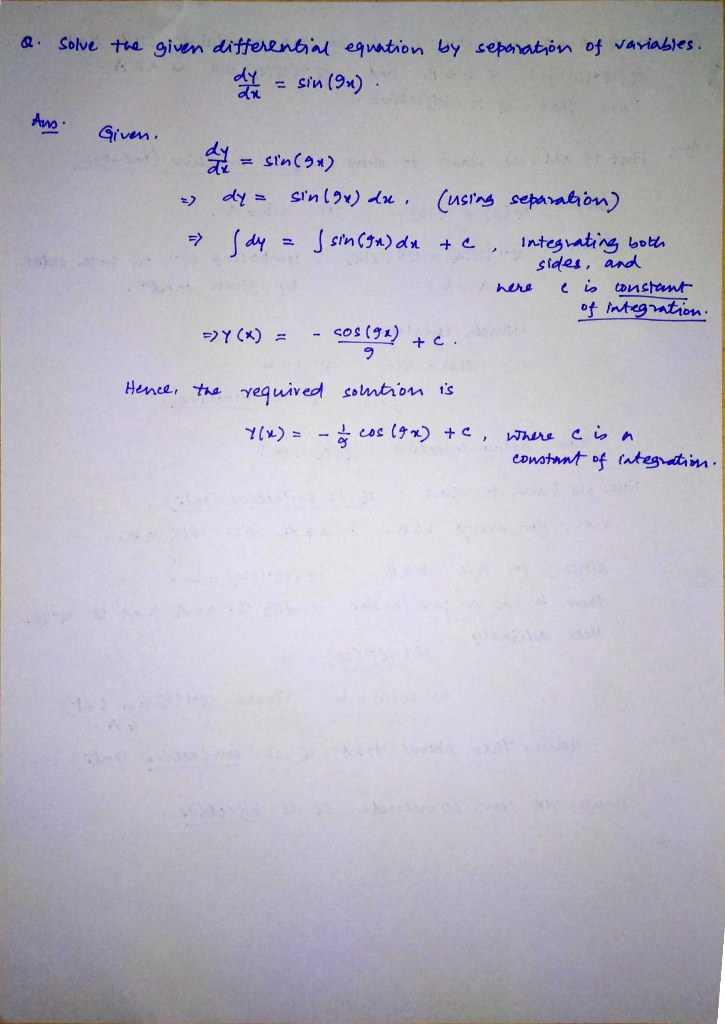#### Earn Coin

Coins can be redeemed for fabulous gifts.

Similar Homework Help Questions
• ### Solve the given differential equation by separation of variables. dy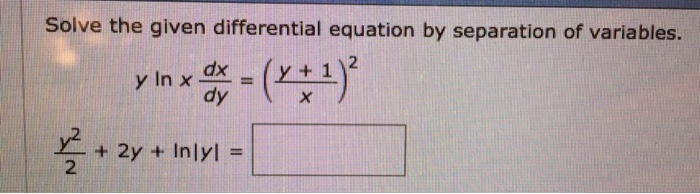Solve the given differential equation by separation of variables. dy

• ### Solve the given differential equation by separation of variables.

Solve the given differential equation by separation of variables. exy(dy/dx) = e-y + e-4x-y

• ### Solve the given differential equation by separation of variables.

Solve the given differential equation by separation of variables. (ey + 1)2e-y dx + (ex + 1)8e-x dy = 0

• ### Solve the given differential equation by separation of variables. dx = (*** 5) 2y + 5)2...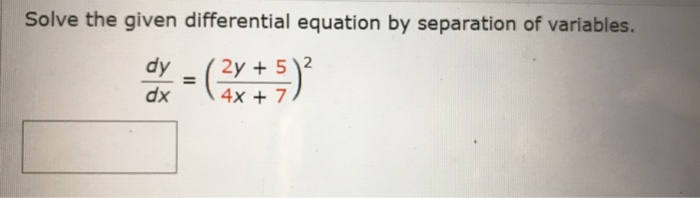Solve the given differential equation by separation of variables. dx = (*** 5) 2y + 5)2 4x + 7

• ### Solve the given differential equation by separation of variables.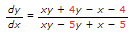Solve the given differential equation by separation of variables. dP/dt= P-P2 Solve the given differential equation by separation of variables. dN/dt + N = Ntet+3 Solve the given differential equation by separation of variables. Find an explicit solution of the given initial-value problem.

• ### please solve the given differential equation 11. [y cos(xy) sin x] dx +xcos(xy) dy 0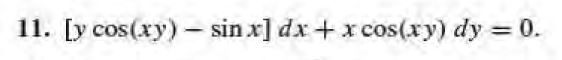please solve the given differential equation 11. [y cos(xy) sin x] dx +xcos(xy) dy 0

• ### solve the following differential equations (e* + 2y)dx + (2x – sin y)dy = 0 xy'...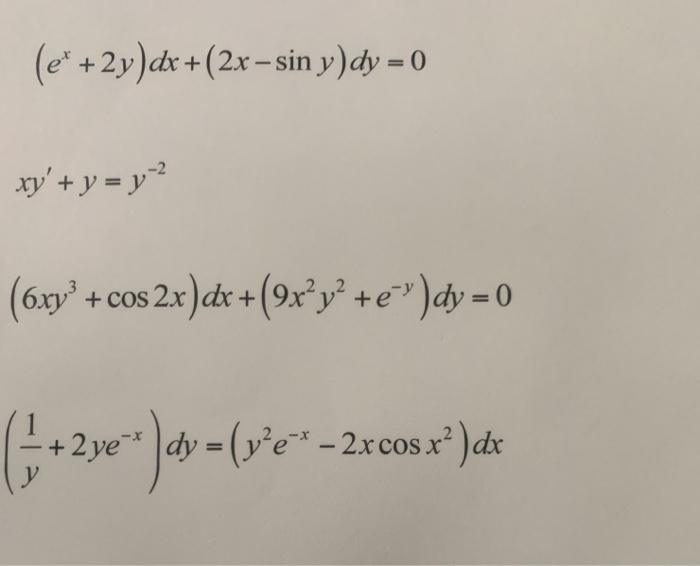solve the following differential equations (e* + 2y)dx + (2x – sin y)dy = 0 xy' + y = y? (6xy + cos2x)dx +(9x?y? +e")dy = 0 +2ye * )dx = (w*e * -2rcos x) di

• ### Solve the given differential equation. (x2 4) dy (2x - 10xy) dx + 1 + 5...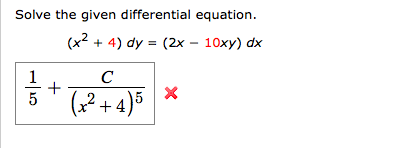Solve the given differential equation. (x2 4) dy (2x - 10xy) dx + 1 + 5 2+4)5 Solve the given differential equation. (x2 4) dy (2x - 10xy) dx + 1 + 5 2+4)5

• ### dy dx Solve the Bernoulli differential equation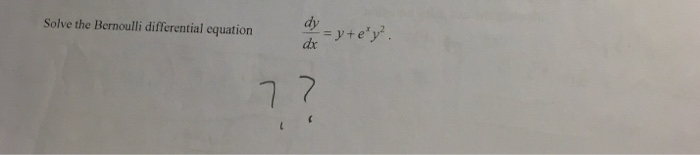dy dx Solve the Bernoulli differential equation

• ### Use the principle of separation of variables to solve dy xyx0 dx Find the constant of...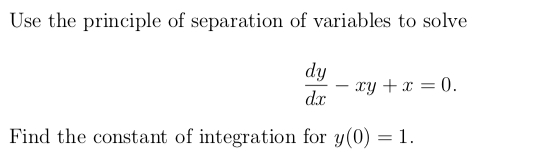Use the principle of separation of variables to solve dy xyx0 dx Find the constant of integration for y(0) = 1.